# Inequalities with one variable inequalities DHS

Definition: an Inequality with a single variable and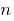two expressions with one variable is United with the sign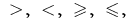which in its General form is written like this:Definition: a Root (or rozvyazka inequalities)is called the value of a variable that turns an inequality into correct numerical equality.

Razvesti inequality means finding all its roots (interchanges) or to show that they are not.

## The area of allowable values of DHS inequality

Definition: the Area of allowable values (range of definition) the bumps — the total scope of functions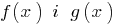standing in the left and right parts of the equation.

### Example

Set the equation: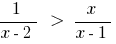DHS: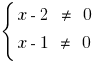i.e.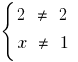you can write and so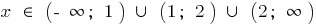.

## Roughness — implications

When rozwiazan roughness effects are not used (and are used is equivalent to conversion), since it is usually impossible to validate all received rozvytku inequalities-consequences.

## Is equivalent to the inequality

Definition: Equivalent to (equivalent to) the bumps two bumps, which for many DHS have the same outcome, that is, every solution of the first inequality is rozvyazka the second, and Vice versa.

### Some theorems about equally dominant either equations

Theorem 1: If one of the inequalities to move to another part of the terms with opposite sign, we get nervst equivalent to a given (on any set).

Theorem 2.1: If both sides of an inequality be multiplied or divided by the same positive integer not equal to zero (or to one and the same function that is defined and not equal to zero on the IDS of the given equation), we get the inequality, tantamount given.

Theorem 2.2: If both sides of an inequality be multiplied or divided by the same VMA integer not equal to zero (or to one and the same function, which is defined and WMA on the odz of the given equation), and KRM in order to change the inequality sign to the opposite, we get the inequality, tantamount given.

Theorem 3.1: If both sidestake increasing function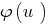(preserving the sign of the inequality) and there is no narrowing of the DHS - specified inequality, the resulting inequalityto be equivalent to the specified ( DHS).

Theorem 3:2 If both sidestake the spotlight function ofchanging the sign of inequalities, and there is no narrowing of the DHS - specified inequality, the resulting inequality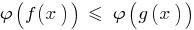to be equivalent to the specified ( DHS).

## Corollaries of theorems about equally dominant either inequalities

Consequence: Because the function ismonotonically increasing,then.

In the presentation of both parts to odd natural degree of the resulting inequality, tantamount given.

Consequence: Because the function is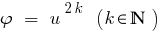monotonically increasing only if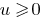,in the case when both sides of an inequality newmn, by lifting both parts of the natural to the even degree get Nernst equivalent to this.

### Example 1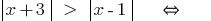(both parts newmn!)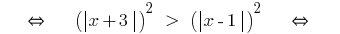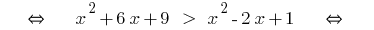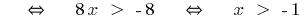Tags:
Chapter:
Versions in other languages:
Share with friends: